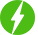# Fundamental Concepts of Finite Element Analysis

0

Fundamental Concepts of Finite Element Analysis, Introduction of FEA Terminology, Introduction to FEA basic concept, Rayleigh’s & Galerkin’s Approach, Types of analysis.

## Course Description

The finite element analysis is a numerical method for solving problems of engineering and mathematical physics. Finite element procedure are at present widely used in engineering analysis It is used to solve Physical problems governed by a differential equation or energy theorem. But this method gives approximate solution. In this mathematical representation and approximate assumptions are made for problem. Applicable even if physical prototype not available and for complicated problems also.

FEA is numerical technique to handle complex geometry, any material properties, any boundary conditions and any loading conditions. Mathematical model of any geometric model describes the behavior of geometry by differential equations and boundary conditions. Mathematical models dividing the object of interest into finite number of elements. FEM is a particular numerical method for solving partial differential equations in two or three space variables (i.e., some boundary value problems). To solve a problem, the FEM subdivides a large system into smaller, simpler parts that are called Finite elements.

The course deals with problems from the area which includes structural analysis, heat transfer, fluid flow, mass transport, electromagnetic potential, topology optimization, modal analysis, transient analysis etc. after completion of this course student are able to explain fundamentals of FEA, terminology in FEA , Rayleigh’s and Galerkin’s Method and Types of analysis

We will be happy to hear your thoughtsRegister New Account
• Total (0)
0